# ELECTRIC FIELD STRENGTH ELECTRIC FIELD Physicists did not

• Slides: 19ELECTRIC FIELD STRENGTH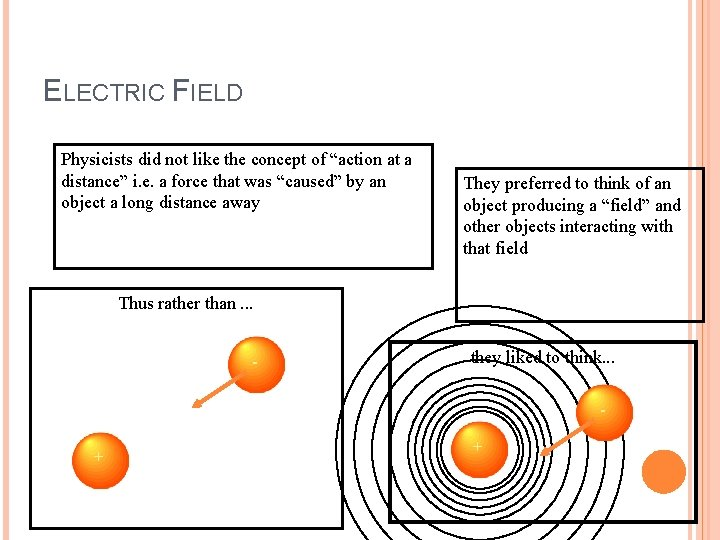ELECTRIC FIELD Physicists did not like the concept of “action at a distance” i. e. a force that was “caused” by an object a long distance away They preferred to think of an object producing a “field” and other objects interacting with that field Thus rather than. . . - they liked to think. . . - + +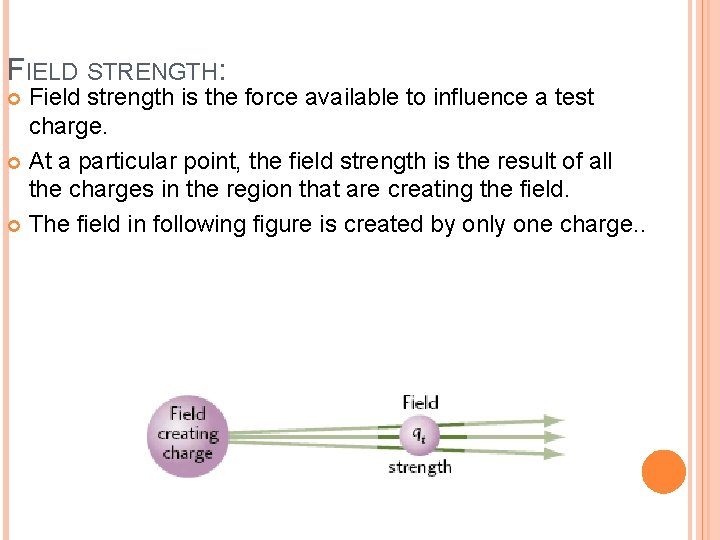FIELD STRENGTH: Field strength is the force available to influence a test charge. At a particular point, the field strength is the result of all the charges in the region that are creating the field. The field in following figure is created by only one charge. .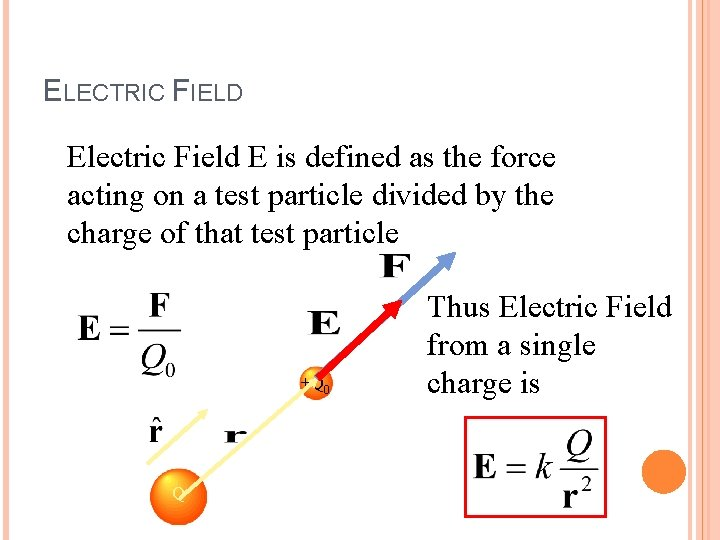ELECTRIC FIELD Electric Field E is defined as the force acting on a test particle divided by the charge of that test particle +Q 0 Q Thus Electric Field from a single charge is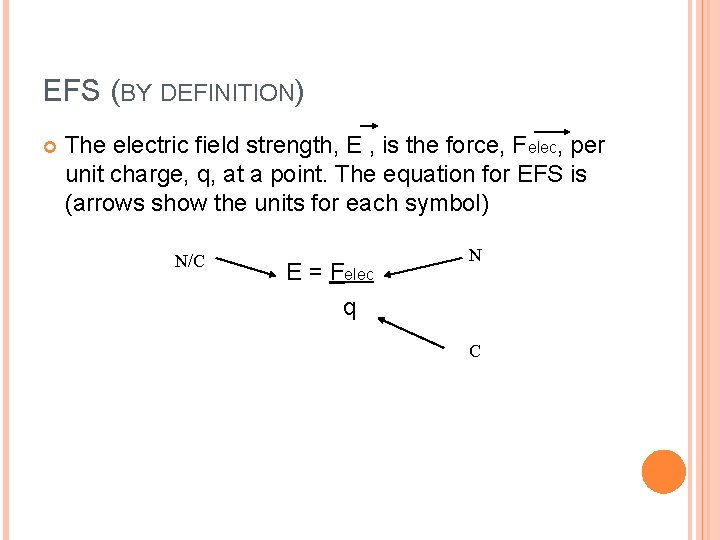EFS (BY DEFINITION) The electric field strength, E , is the force, Felec, per unit charge, q, at a point. The equation for EFS is (arrows show the units for each symbol) N/C E = Felec q N C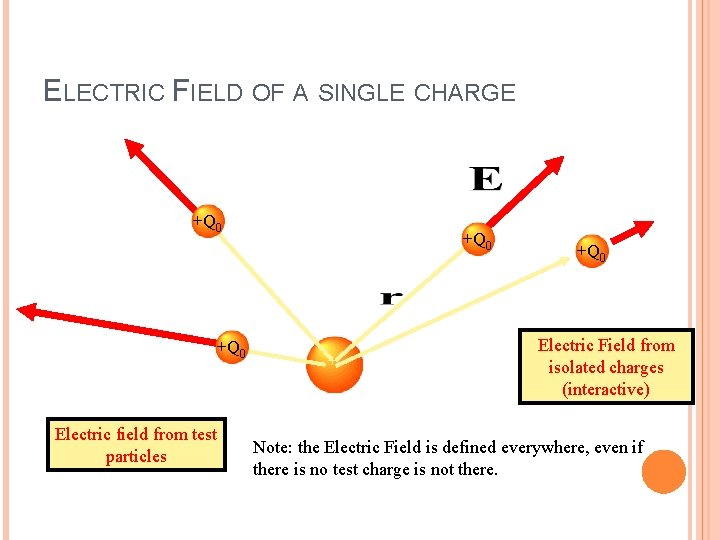ELECTRIC FIELD OF A SINGLE CHARGE +Q 0 Electric field from test particles +Q 0 + +Q 0 Electric Field from isolated charges (interactive) Note: the Electric Field is defined everywhere, even if there is no test charge is not there.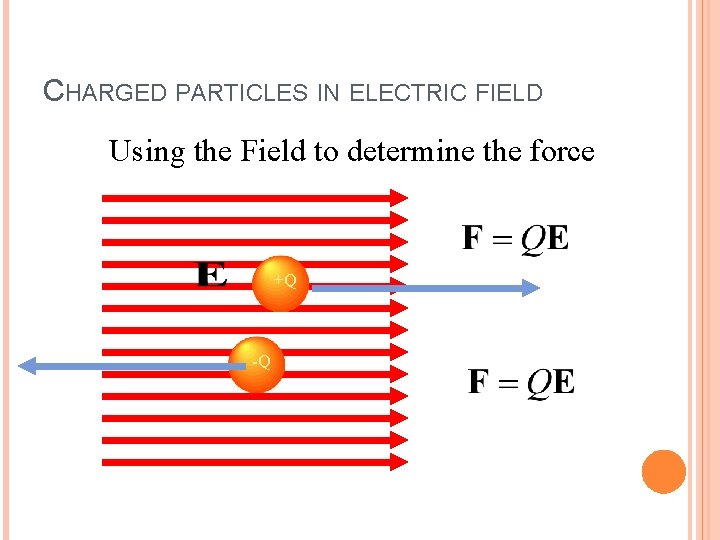CHARGED PARTICLES IN ELECTRIC FIELD Using the Field to determine the force +Q -Q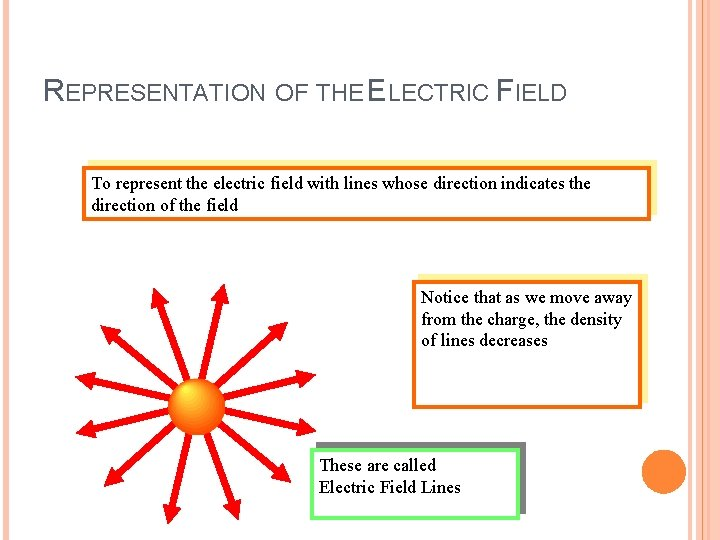REPRESENTATION OF THE ELECTRIC FIELD To represent the electric field with lines whose direction indicates the direction of the field Notice that as we move away from the charge, the density of lines decreases These are called Electric Field Lines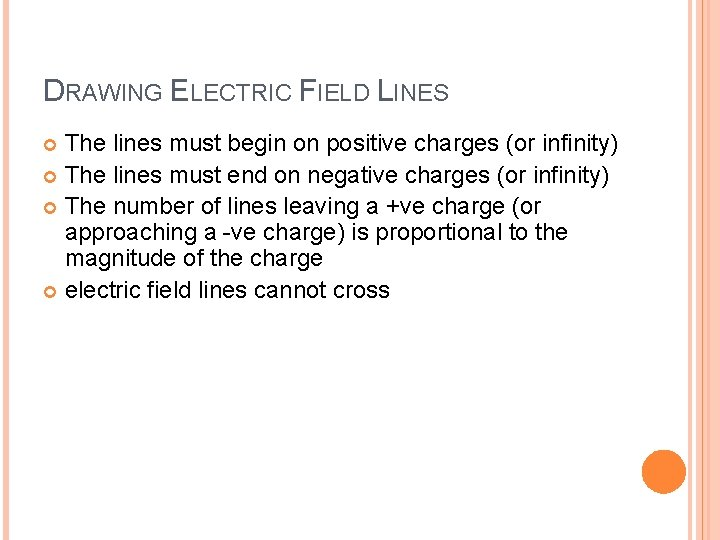DRAWING ELECTRIC FIELD LINES The lines must begin on positive charges (or infinity) The lines must end on negative charges (or infinity) The number of lines leaving a +ve charge (or approaching a -ve charge) is proportional to the magnitude of the charge electric field lines cannot cross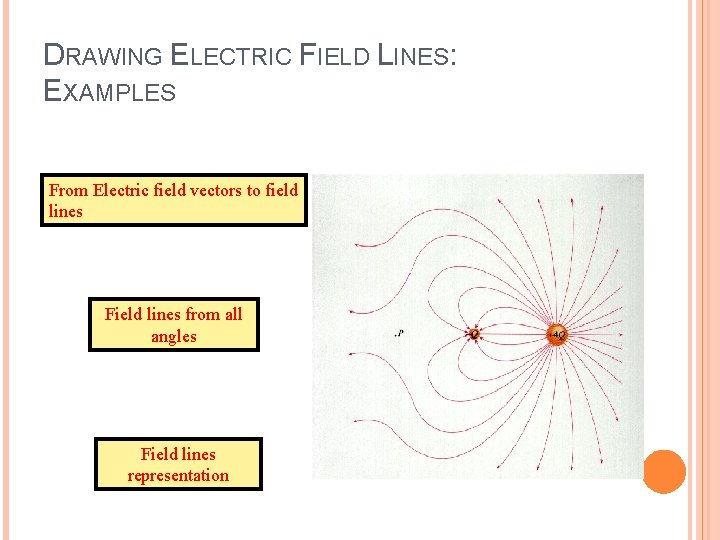DRAWING ELECTRIC FIELD LINES: EXAMPLES From Electric field vectors to field lines Field lines from all angles Field lines representationELECTRIC FIELD STRENGTH 1. 2. 3. Steps to measure EFS, E, at some point. Place small + test charge (q) at that point Measure the force (Felec) exerted on the test charge Divide force by the size (amt. ) of test charge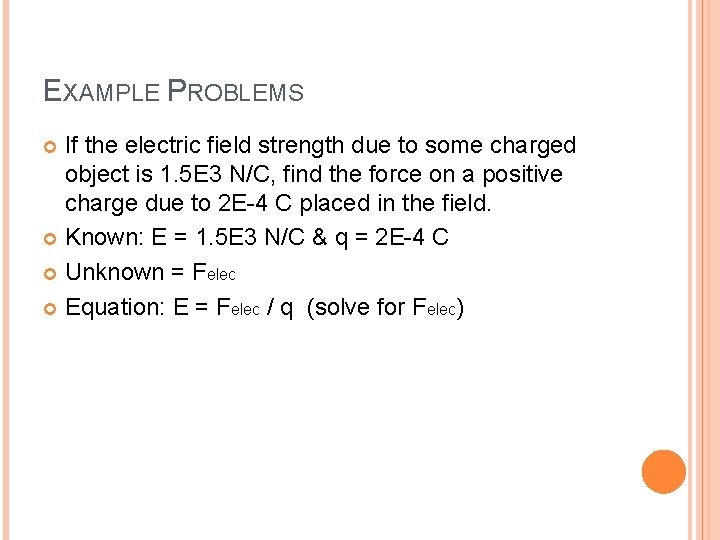EXAMPLE PROBLEMS If the electric field strength due to some charged object is 1. 5 E 3 N/C, find the force on a positive charge due to 2 E-4 C placed in the field. Known: E = 1. 5 E 3 N/C & q = 2 E-4 C Unknown = Felec Equation: E = Felec / q (solve for Felec)EXAMPLE PROBLEMS (CONT. ) Felec = (1. 5 E 3 N/C) x (2 E-4 C) = 0. 30 N If the charge on the object in example #1 was doubled, does that change the electric field strength? Hint: what change would increasing q have on Felec?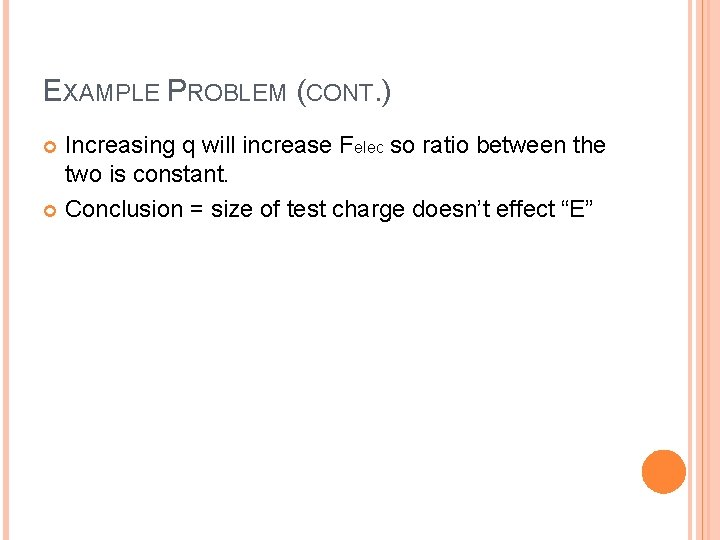EXAMPLE PROBLEM (CONT. ) Increasing q will increase Felec so ratio between the two is constant. Conclusion = size of test charge doesn’t effect “E”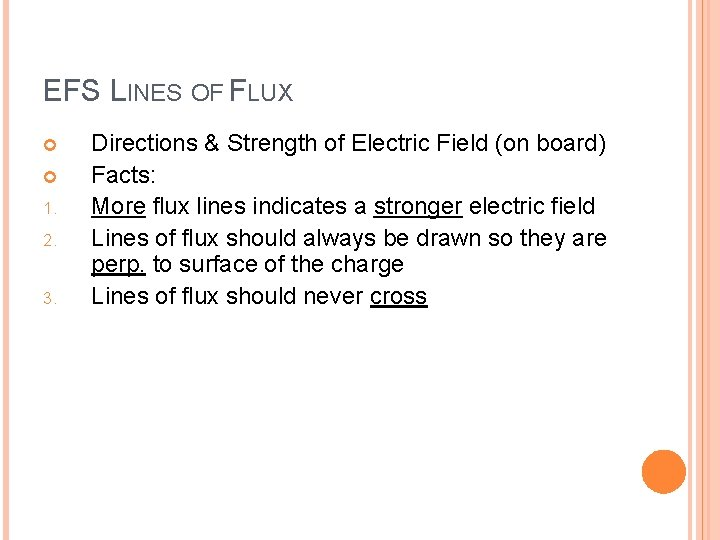EFS LINES OF FLUX 1. 2. 3. Directions & Strength of Electric Field (on board) Facts: More flux lines indicates a stronger electric field Lines of flux should always be drawn so they are perp. to surface of the charge Lines of flux should never cross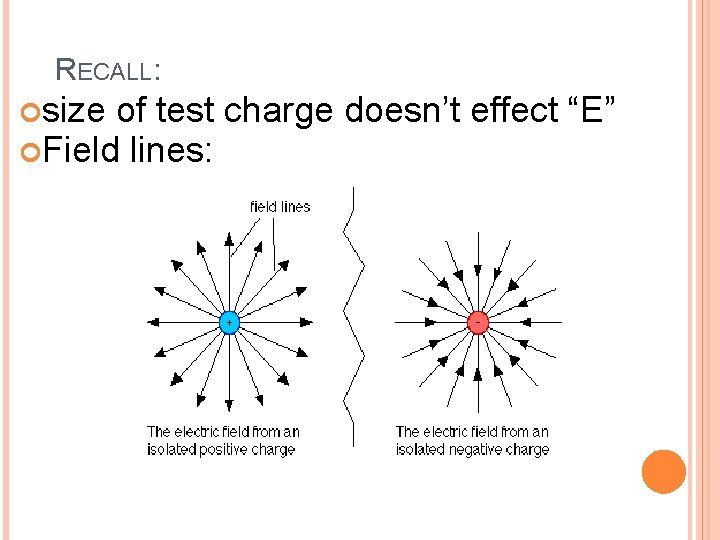RECALL: size of test charge doesn’t effect “E” Field lines: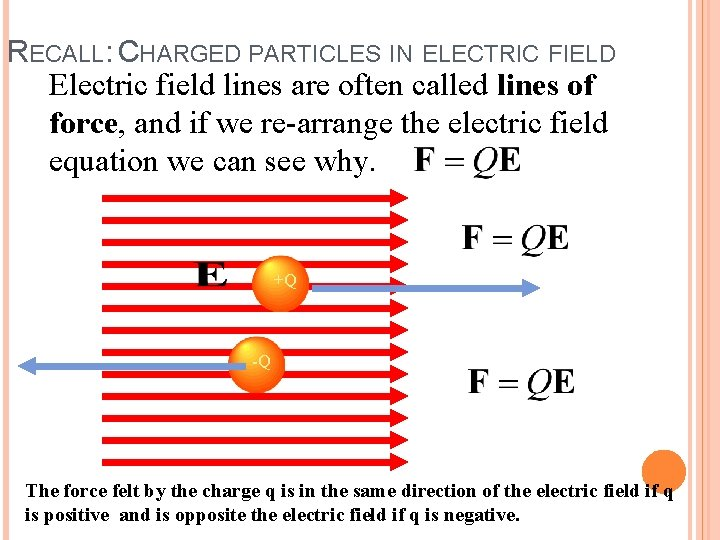RECALL: CHARGED PARTICLES IN ELECTRIC FIELD Electric field lines are often called lines of force, and if we re-arrange the electric field equation we can see why. +Q -Q The force felt by the charge q is in the same direction of the electric field if q is positive and is opposite the electric field if q is negative.To represent the strength of the electric field, the number of lines that enter or leave a charge are proportional to the magnitude of the charge. If Q 1 is 1 μC and Q 2 is -2μC then half as many field lines leave _______ as enter ____. Q 1 Q 2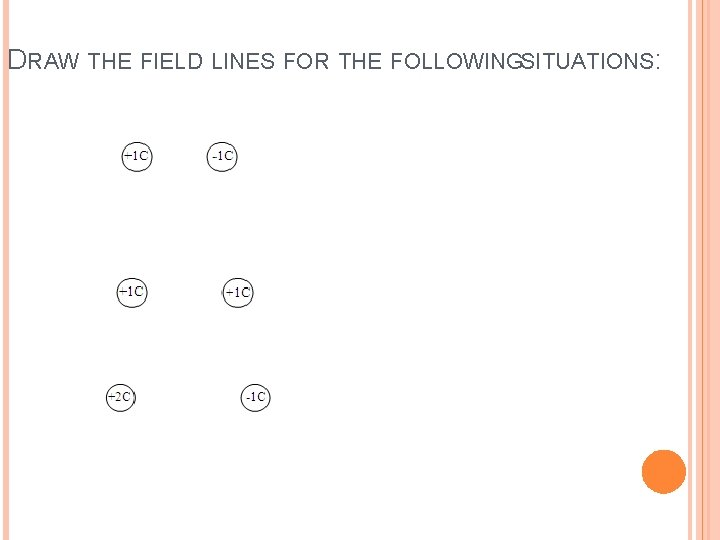DRAW THE FIELD LINES FOR THE FOLLOWINGSITUATIONS: